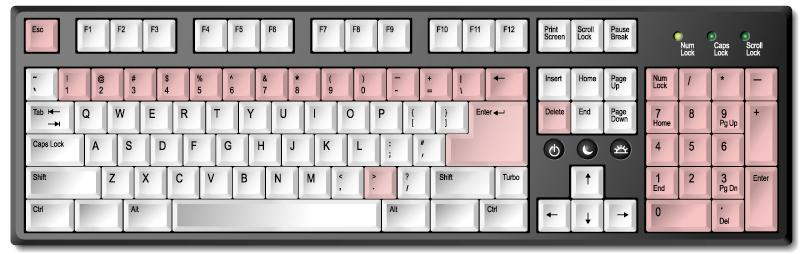# Simple calculator - fast, accurate

A simple electronic calculator online produces a series of simple mathematical calculations. The kalkpro.ru online calculator is an effective and more practical replacement for the standard solution from Microsoft (installed calculator in Windows on your computer).

The calculator is available for free and at any time is ready to provide the most accurate calculations in a short period of time. Why is kalkpro.ru the most convenient and accurate? Read below!

## The advantages of simple calculator kalkpro.ru

Compared to other online calculators, our solution makes the calculations accurate to 20 decimal places. Normal services break down up to 10 characters. It is connected with necessity of large computational resources. To display up to 30 characters, required equipment with enormous productivity. Our service has the following advantages:

• the scale calculator at a convenient size;
• adaptation to any size of the display;
• calculations are made according to the rules of mathematics taking into account the priority of transactions of different type (addition, multiplication, etc.);
• the most accurate calculations;
• costing the entire expression at once, not in parts.

Math calculator lets you calculate any of the values according to the canons of mathematical laws. Creates subtotals after entering each action, making a calculation taking into account all the mathematical expressions, and not all operations separately.

## Calculation example

If we try to determine the result of calculations the following example, 3+3x3, then the system will give the result 12. Other calculators, as a rule, will give the result 18. This is due to the fact that after each action is determined by bodytag. Our program takes into account the hierarchy of mathematical symbols, performing a first multiplication operation and then addition.

## How to count on a simple calculator

Simple calculator is a simple calculation to estimate amounts or the difference, to perform the operations of division and multiplication. When entering values, use the mouse or keyboard keys. The most convenient for calculations is a digital unit, but you can use those keyboard buttons that are between functional and next to a literal block. All numeric values on the keypad correspond to the buttons on the online calculator.For the implementation of addition and multiplication, division and subtraction using the following keys on the PC keyboard:

[*] - multiply;

[/] - divide;

[-] - subtract;

[Enter] the same;

[.] - decimal separator.

To know the result of a mathematical operation, you must press [Enter] on the keyboard or the sign [=] the calculator interface. To reset the values using the keyboard, press one of the keys [Del] or [Esc]. Pressing [Backspace] to delete the last entered value.

### What operations can be done on a simple calculator?

• (x) – multiplication;
• ( ÷ ) division;
• (-) – subtract;